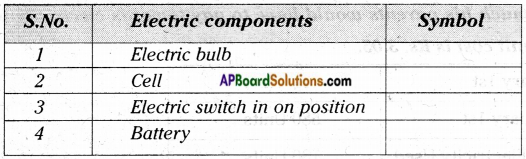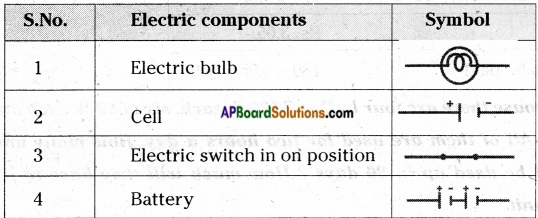AP State Syllabus AP Board 7th Class Science Important Questions Chapter 7 Electricity – Current and Its Effect

## AP State Syllabus 7th Class Science Important Questions 7th Lesson Electricity – Current and Its Effect

### 7th Class Science 7th Lesson Electricity – Current and Its Effect Important Questions and Answers

Question 1.
What are Series and Parallel electric circuits?
A) Series circuits:

1. In a series circuit, electricity has only one path to flow through.
2. All the electrical components are connected in this path.
3. If any one of them is removed or is not functioning properly, the circuit will be incomplete.

B) Parallel circuits:

1. A parallel circuit has more than one path for the flow of electricity.
2. Each bulb in the circuit is connected in a separate path through which electricity can flow.Question 2.
Describe the effect of connecting electrical cells in series. How would you connect them in series?
Connecting Electrical cells in series:

1. Take a dry cell and torch bulb.
2. Connect the bulb to a cell using copper wires shown in figure-a. Observe the intensity of light.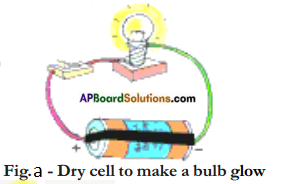3. Now take one more dry cell and connect two cells as shown in figure-b.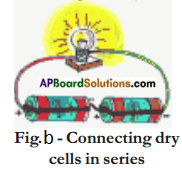4. In this method the positive terminal of the first cell and the negative terminal of the second cell are connected to the bulb.
5. .When the cells are connected in series more electricity flows in the circuit and the bulb glows with greater brightness.

Question 3.
Describe the connection of cells in parallel. Draw the circuit diagram also.
Connecting Electric Cells in Parallel:

1. Take three dry cells and connect them as shown in figure-a.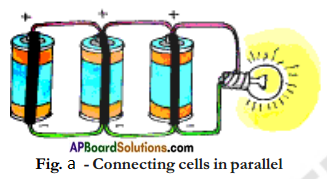2. That is all the positive terminals of the three cells are connected together and all the three negative terminals are connected together.
3. These three positives and three negatives are connected together to a bulb.
4. There is no difference in the intensity of the bulb glow compared to that in the case of only one cell.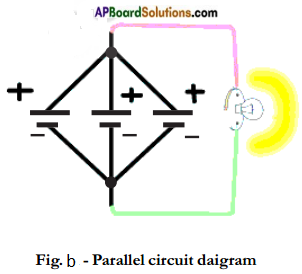Question 4.
How are electric bulbs connected in series? Where do you find such connections in your daily life? What is the disadvantage with this arrangement?
Connecting electric bulbs in series:

1. Connect three torch bulbs in series as shown in figure.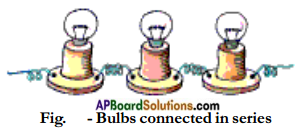2. Connect this to a dry cell. Observe the brightness of each of the three bulbs.
3. Now connect one more dry cell in series with the first cell.
4. Observe the brightness of each of the bulb.
5. Then connect one more dry cell in series with the first two cells. Again observe the bulbs. The brightness of the bulbs increases with the addition of cells.
6. In series connection of bulbs, if one bulb gets fused, all the other bulbs in the series will stop glowing.
7. It means that if one bulb is disconnected the other bulbs do not glow. This is the disadvantage.
8. This can be observed in serial bulbs used in decorative items at the time of marriages and other festivals.

Question 5.
a) How are electric bulbs connected in parallel?
b) What is, the advantage with this type of connection?
c) Where do you find such connections?
Connecting bulbs in parallel:
a)

1. Connect three bulbs in parallel as shown in figure.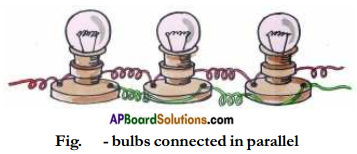2. That is, one end of each of the three bulbs are connected to one wire.
3. The other ends of the three bulbs are connected to another wire.
4. These two wires are connected to a cell. All the three bulbs glow dimly.
5. Now disconnect one of the bulbs.

b)

1. It means that if one bulb is disconnected the other bulbs continue to glow.
2. This is the advantage with this type of connection.
3. This can be observed in our household electric circuit. All components in our houses are connected in parallel.Question 6.
What are the advantages of using tube lights and compact fluorescent lamps.

1. Wastage of electricity can be reduced by using fluorescent tube lights in place of the bulbs, as shown in figure, (a).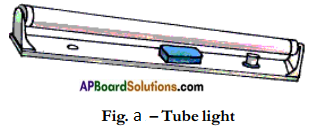2. Compact Fluorescent Lamp (CFLs) shown in figure, (b) also reduces wastage and can be fixed in ordinary bulb holders.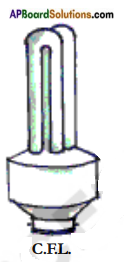3. The ISI mark of a lamp ensures that the appliance is safe.

Question 7.
What is an electric fuse? Explain its importance. How does it work?
Electric Fuses:

1. When excessive electric current flows through a circuit the wires or the appliances may get heated and can catch fire.
2. To avoid fire, a safety device known as a fuse is connected in series in the circuit.
3. A fuse is a small piece of wire as shown in figure.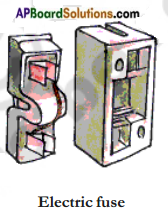4. It is made of a special alloy that gets heated quickly and melts.
5. If the current in the circuit is too high, the fuse wire gets hot and melts.
6. This leaves a gap in the circuit. Automatically the circuit is broken and flow of electricity is stopped.
7. This protects appliances from getting burnt due to the passage of too large a current through them.Question 8.
What is a Miniature Circuit Breaker (MCB)? What is its advantage? For which mark should we look at it?
Miniature Circuit Breaker (MCB):

1. These days Miniature Circuit Breaker (MCB) is increasingly being used in place of fuses.
2. These are switches which automatically turn off when current in a circuit exceeds the safe limit.
3. It has a switch which goes OFF automatically if there is overheating.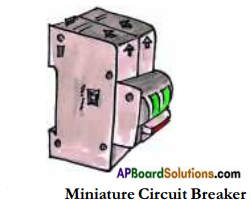4. This breaks the circuit. If we turn them on, the circuit is once Miniature Circuit again complete.
5. The advantage miniature circuit breakers have over fuses is that they can be reset (manually or automatically) to restore normal operation, whereas fuses need to be replaced after every single operation.
6. The MCB can be reset by hand and the circuit becomes complete once again.
7. Look for ISI mark on MCBs.

Question 9.
What are the inventions of Michael Faraday?
Michael Faraday (1791-1867): Michael Faraday observed that by moving a magnet in and out of a coil we can make electric current flow through the coil. Using this he built the first electric generator or dynamo in 1831. He also invented the transformer.

Question 10.
What is the importance of symbols? What role do they play in our life?

1. We know about symbols. We do indicate to our teacher that we wish to go out to drink water.
2. We know the signs for addition, subtraction, multiplication and division.
3. We might have used the symbols for ‘greater than’, ‘less than’, ‘equal to’ etc.
4. Symbols play an important role in our life.
5. They convey precise meaning with few descriptions.
6. Some common electric components can be represented by standard symbols.

Question 11.
What happens to the electric bulb when the current is passed?

1. The bulb becomes hot when we put it on for some time.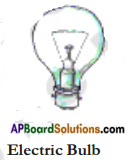2. It is the filament of the bulb that heats up due to current flowing through it.Question 12.
What do you understand by the term ‘the filament of an electrical appliance’?

1. We have seen an electric iron, electric cooker and electric heater.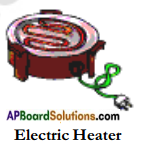2. All these contain a coil of wire made up of Nichrome.
3. This coil is called a filament of the appliance.

Question 13.
What happens when electrical appliances like electric iron, electric heater etc., are switched on?

1. We have noticed that when these appliances electric heater, electric iron are switched on, their filaments become red hot and give out heat.
2. The amount of heat produced in a wire depends on its materials, length and thickness.
3. Thus, for different requirements, the wires of different materials, lengths and thicknesses are used.

Question 14.
What happens when an electric current passes through wires used for making electric circuits and through the elements of some electric appliances?

1. The wires used for making electric circuits do not normally become hot.
2. On the other hand, the elements of some electric appliances become so hot that they are easily visible.
3. The filament of an electric bulb gets heated to such a high temperature that is starts glowing and giving out light.

Question 15.
Describe how can you make your own cell. Explain how do you test the working condition of that cell.

1. We will need a few things to make a cell.
2. First get two injection bottles. Then cut two 3 cm long bits of thick copper wire. Use sandpaper to scrape about 1 cm of the coating off both ends of the wires.
3. Break open a discharged dry cell and remove its outer metal covering (made of Zinc). Cut two 2 mm wide and 3 cm long strips from this zinc plate.
4. Insert the copper wires and zinc strips separately into the rubber caps of the injection bottles as shown in Fig.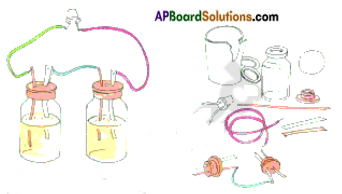5. Ensure that the copper wires and zinc strips do not touch each other.
6. Now take a wire and connect the zinc plate of one bottle with the copper wire of the other bottle.
7. Fill both bottles with sulphuric acid. Carefully close the bottles with the caps in which the copper wires and zinc strips are inserted.
8. The cell is ready. To test its working take a LED.
9. Attach two wires to its two terminals. Touch the wire from one terminal to the copper wire of the first bottle and the wire from the other terminal to the zinc plate of the second bottle.
10. The LED lights up. It means the cell is working.Question 16.
What can you see inside a dry cell when it is cut open?

1. With the help of your teacher cut open a dry cell.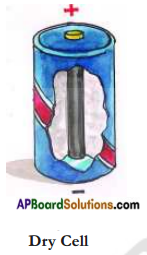2. Observe the chemical components in the dry cell.
3. Inside a dry cell there are certain chemicals which react with one another to produce electric energy.

Question 17.
Think of the main use of electrical appliances and write their names in a table.

Question 18.
How is electricity supplied to our houses ? Are we getting it free of cost?

1. Most of the electricity that we use at home and at school is alternating current.
2. The main electricity that is supplied to our houses comes from power stations and sub-stations distribute electricity.
3. Electricity is not free of cost.
4. We have to pay for it according to how much we consume.

Question 19.
How can you know the current consumed in your house?

1. We notice that a person belonging to the electricity department, the meter reader, visits houses every month and takes the meter readings.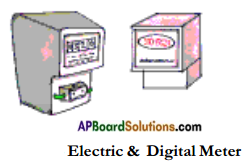2. If we look at the meter in our house we will notice a wheel that goes around and the numbers in the window keep changing.
3. Modern meters have digital displays.

Question 20.
What does the term ’one unit’ mean? How is the usage of electricity measured?

1. If we look at the bulbs used in our home, we will notice that they are marked in watts 25 W, 40W, 60W, 100W.
2. The wattage measures how ‘powerful’ the bulb is.
3. The brighter the bulb, the higher its wattage and the more the electricity used by it.
4. 1 kilowatt (KW) is 1000 watts (W).
5. When any appliance of 1 Kilo Watt is used for one hour, it uses up one Kilo Watt – hour (KWH) or ‘one unit’ of electricity.
6. If it runs for two hours it will use up two units of electricity.Question 21.
Draw a neat diagram of the dry cell and label its parts.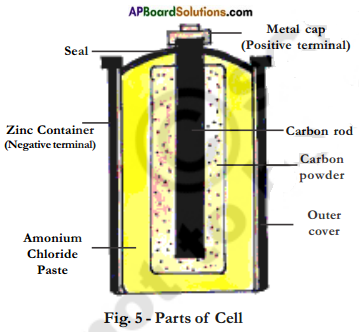Question 22.
Describe the construction of a dry cell.
Description of a dry cell:

1. A dry cell consists of a container made up of zinc metal.
2. The container also serves as the negative terminal in the centre.
3. A carbon (graphite) rod with a metal cap serves as a positive terminal.
4. The carbon rod is surrounded by a mixture of carbon particles and a chemical called ammonium chloride.
5. The cell is sealed from the top.
6. The dry cell can supply electric current in a circuit for a certain time.
7. After that/its chemicals get exhausted and it cannot be used any more.
8. Dry cell converts chemical energy into electrical energy.

Question 23.
Write the symbols of some common electric components and describe their use.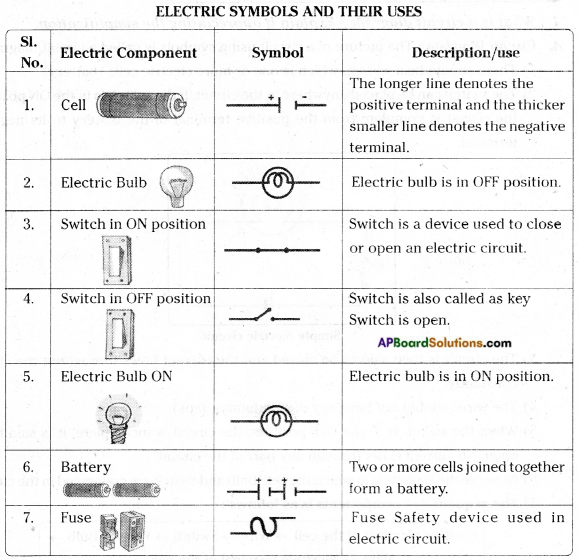Question 24.
Draw a simple switch to close the circuit. For that draw the circuit diagram.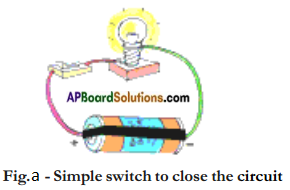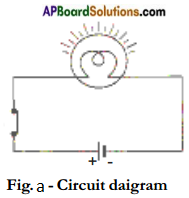1. See figure (a). A bulb, battery and switch are connected as shown.
2. Can we make this drawing simpler using symbols ?
3. The picture of circuit using symbols is called a circuit diagram.
4. Figure (b) shows a circuit diagram of the same circuit as shown in figure (a).

Question 25.
What is a circuit diagram? Explain it appreciating the simplification.
Circuit Diagram: The picture of a circuit using symbols is called a circuit diagram.

1. There should be a source, which is one or more electric cells (battery).
2. The switch can be placed anywhere in the circuit. If the switch is in the ON position, the circuit is complete from the positive terminal of the battery to its negative terminal.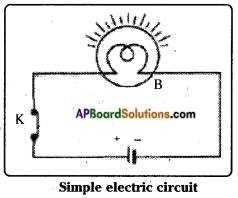3. The circuit is then said to be closed and the current flows throughout the circuit constantly.
4. The wires should not have any discontinuity (gaps).
5. When the switch is in the OFF position, the circuit is incomplete. It is said to be open. No current flows through any part of the circuit.
6. Observe the sequence in which the cell, bulb and switch are connected in the circuit.
7. The sequence of components is as follows:
Positive terminal of the cell → Wire → Switch → Wire → Bulb → Wire → Negative terminal of the cell

Question 26.
Why does the bulb glow brighter and brighter when electric cells are connected in series?

1. The electricity can flow through one path when electric cells are connected in series.
2. Addition of a cell in such a circuit adds strength to the electricity flowing.
3. As more electricity flows into the bulb its glow becomes brighter.

Question 27.
Does electric bulb in your house glow with dry cell? Why?

1. The electric bulb in our house does not glow with dry cell.
2. The electric bulb in our houses glows with the supply of A.C. at high voltage.
3. Dry cell supplies only D.C. at a low voltage.

Question 28.
Are the cells used in a torch light and wrist watch the same?
They are not the same.Question 29.
What is the reason for connecting electric bulbs in parallel in household electric appliances?

1. In the house every bulb should glow independently without depending upon the working condition of other bulbs.
2. This is possible only with parallel connection.
3. So electric bulbs or electric appliances are connected in parallel in household electric connections.

Question 30.
How do we get a battery?
By connecting small cells in series we get a battery.

Question 31.
The meter reading in Ayub’s house in January is 400 units, February 580 units. Calculate how much his parents would have to pay towards electricity bill of February. The unit cost is Rs. 3.05.

 Reading on January 1st 400 Units Reading on February 1st 580 Units Number of units Electricity Used 180 Units Cost per unit Rs. 3.05/- Total Amount to be paid 180 × 3.05 = 549/­

Question 32.
Suppose in a house there are four bulbs of 100 W each, six of 60 W each and six of 40 W each. All of them are used for two hours a day. How many units of electricity will be used up in 30 days? How much will they have to pay at Rs. 2.80/- per unit.

1. Total power used = (4 × 100 W) + (6 × 60 W) + (6 × 40 W) = 1000 W = 1 KW
2. Total power used every day = 2 hrs × 1 KW = 2 KWH
In 30 days, power used = 2 × 30 KWH = 60 KWH
3. The cost of the power is = Rs. 2.80 × 60 = Rs. 168/-

Question 33.
Why should we use electricity carefully and only when needed?

1. Our country faces a shortage of electricity.
2. So wasting electricity means we are depriving someone else of electricity.
3. Our bill also goes up.
4. So use electricity carefully and only when it is needed.Question 34.
a) Look at the diagram, correct it and redraw.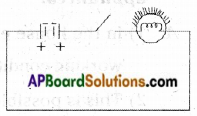b) Guess the reasons why the bulb did not glow in the circuit.
a)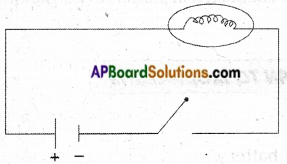b)

1. The wires are not properly connected.
2. The battery lost its efficiency
3. The battery is not set properly.
4. The bulb has lost its filament.

Question 35.
Fill the table with suitable symbol of electric components listed in the table.